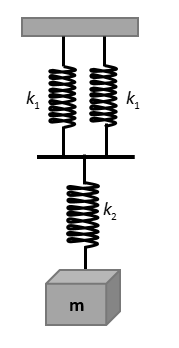Courses
Courses for Kids
Free study material
Free LIVE classes
More# What will be the force constant of the spring system shown in the figure,Fig: The spring mass systemA. $\dfrac{{{k_1}}}{2} + {k_2}$B. ${\left[ {\dfrac{1}{{2{k_1}}} + \dfrac{1}{{{k_2}}}} \right]^{ - 1}}$C. $\dfrac{1}{{2{k_1}}} + \dfrac{1}{{{k_2}}}$D. ${\left[ {\dfrac{2}{{{k_1}}} + \dfrac{1}{{{k_2}}}} \right]^{ - 1}}$Verified
54.3k+ views
Hint: we find the equivalent spring constant of the springs connected in parallel and then the join it in series with other spring. The final combination will be of two springs in series. Then finding the equivalent spring constants we get the final spring constant of the combinations of the given springs.

Formula used:
${K_{eq}} = {k_1} + {k_2} + {k_3} + \ldots + {k_n}$, the formula for the equivalent spring constants when n springs are connected in parallel with spring constants ${k_1},{k_2},{k_3} \ldots \ldots$
$\dfrac{1}{{{K_{eq}}}} = \dfrac{1}{{{k_1}}} + \dfrac{1}{{{k_2}}} + \dfrac{1}{{{k_3}}} + \ldots + \dfrac{1}{{{k_n}}}$, the formula for the equivalent spring constants when n springs are connected in series with spring constants ${k_1},{k_2},{k_3} \ldots \ldots$

Complete step by step solution:Fig: The spring mass system
In the given figure, the springs with spring constants${k_1}$and ${k_1}$ are connected in parallel with each other. So the equivalent of these two springs will be calculated using formula for the equivalent spring constant when the springs are connected in parallel,
Let the equivalent spring constant of the two springs with spring constants${k_1}$and ${k_1}$is $k'$,

Using the formula, we get
$k' = {k_1} + {k_1}$
$k' = 2{k_1}$
Now, the equivalent of the first two springs are in series with the other spring with spring constant ${k_2}$

So, the final spring constant will be equivalent of these two springs will be calculated using formula for the equivalent spring constant when the springs are connected in series,
$\dfrac{1}{{{k_{eq}}}} = \dfrac{1}{{k'}} + \dfrac{1}{{{k_2}}}$
$\dfrac{1}{{{k_{eq}}}} = \dfrac{1}{{2{k_1}}} + \dfrac{1}{{{k_2}}}$
${k_{eq}} = {\left[ {\dfrac{1}{{2{k_1}}} + \dfrac{1}{{{k_2}}}} \right]^{ - 1}}$

So, the equivalent spring constant of the given spring system is ${\left[ {\dfrac{1}{{2{k_1}}} + \dfrac{1}{{{k_2}}}} \right]^{ - 1}}$

Therefore, the correct option is (C).

Note: In case of identification of the springs which are connected in series or parallel, we make small displacement in the block attached, the springs in which the compression is same are in parallel and if the compressions are different then the springs are in series.
Last updated date: 23rd May 2023
Total views: 54.3k
Views today: 0.13k